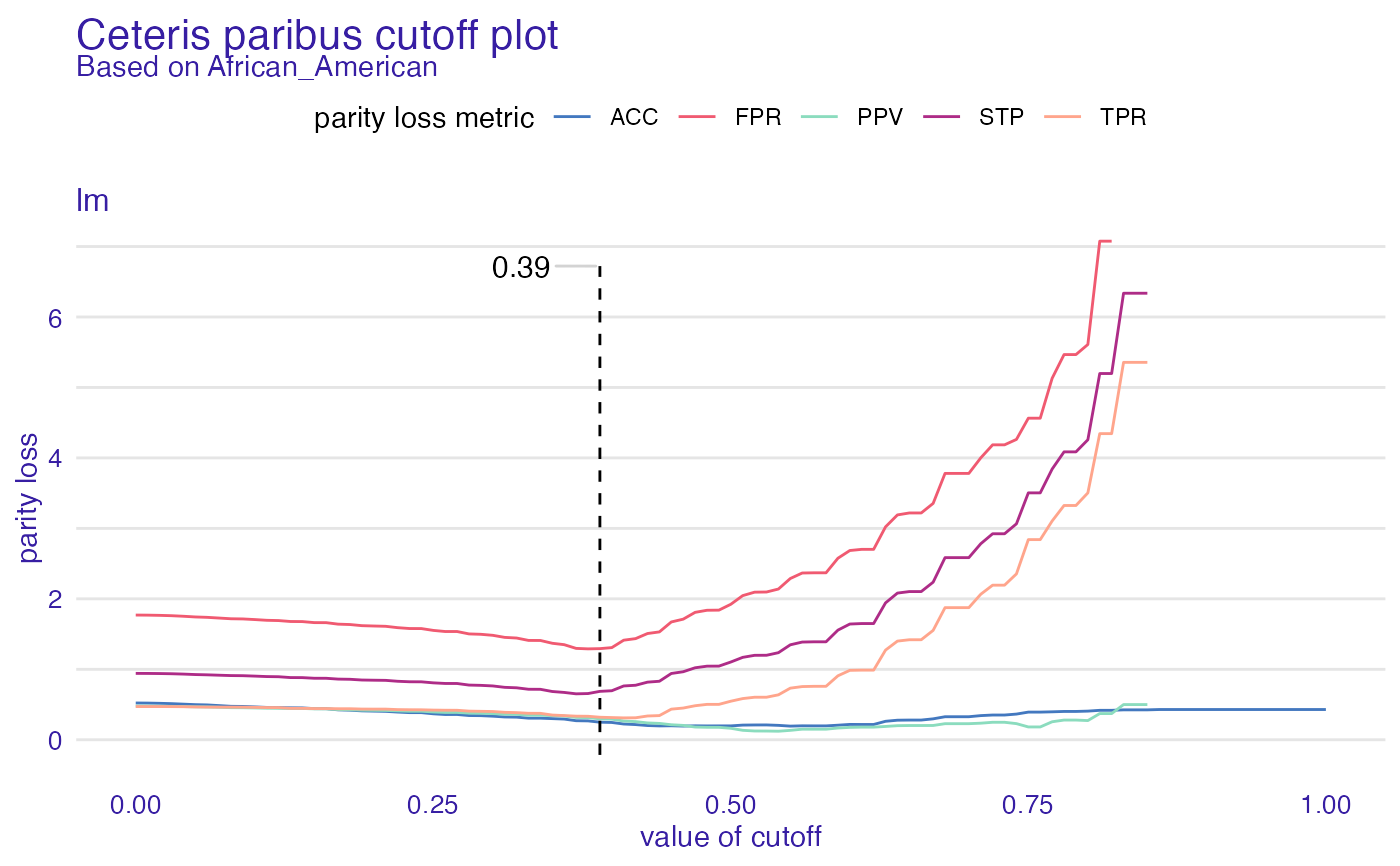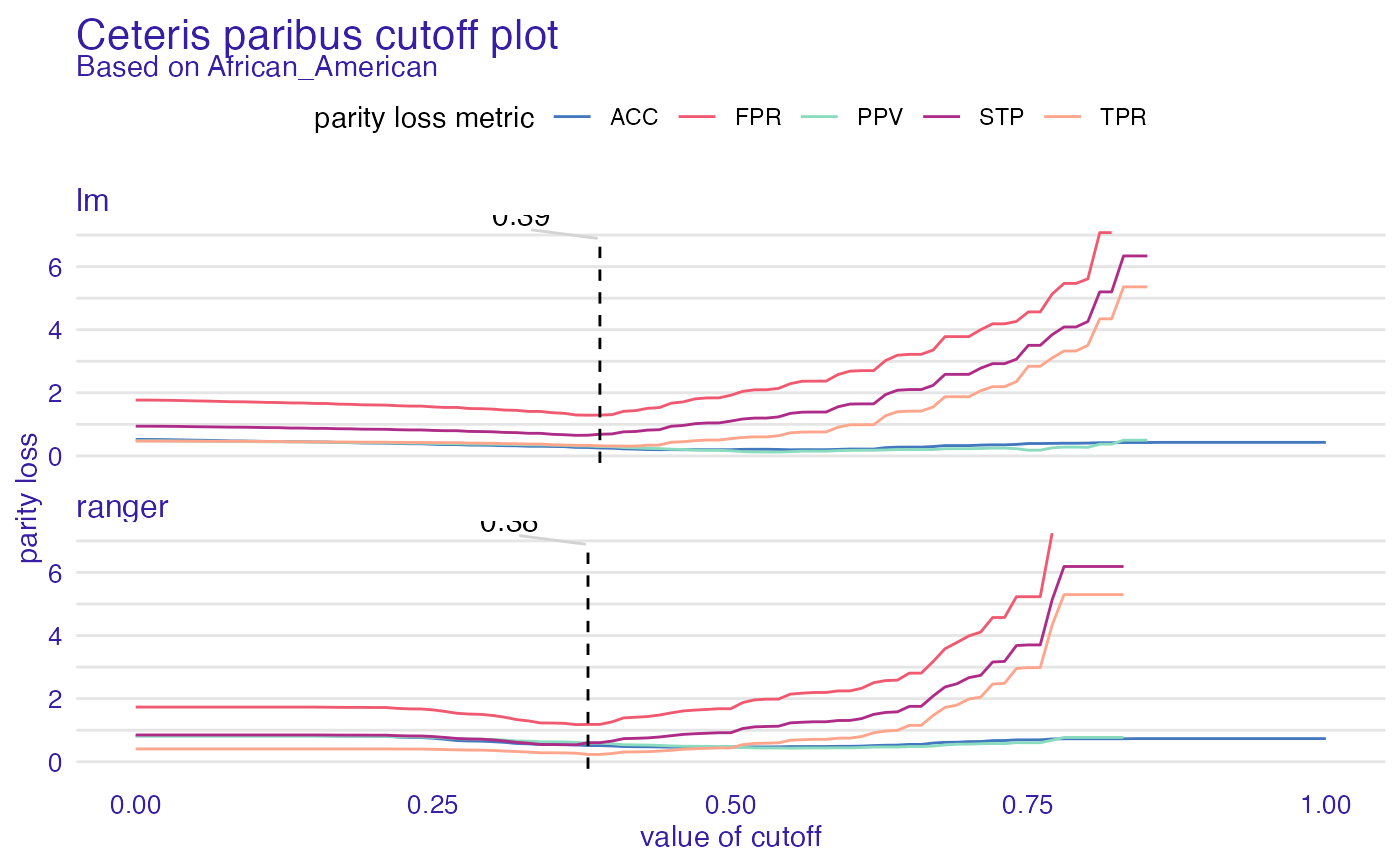Ceteris paribus cutoff is way to check how will parity loss behave if only cutoff for one subgroup was changed. By using parameter new_cutoffs parity loss for metrics with new cutoffs will be calculated. Note that cutoff for subgroup (passed as parameter) will change no matter new_cutoff's value at that position. When parameter cumulated is set to true, all metrics will be summed and facets will collapse to one plot with different models on it. Sometimes due to the fact that some metric might contain NA for all cutoff values, cumulated plot might be present without this model.

ceteris_paribus_cutoff(
x,
subgroup,
new_cutoffs = NULL,
fairness_metrics = c("ACC", "TPR", "PPV", "FPR", "STP"),
grid_points = 101,
cumulated = FALSE
)

## Arguments

x object of class fairness_object character, name of subgroup (level in protected variable) list of cutoffs with names matching those of subgroups. Each value should represent cutoff for particular subgroup. Position corresponding to subgroups in levels will be changed. Default is NULL character, name of parity_loss metric or vector of multiple metrics, for full metric names check fairness_check documentation. numeric, grid for cutoffs to test. Number of points between 0 and 1 spread evenly. logical, if TRUE facets will collapse to one plot and parity loss for each model will be summed. Default FALSE.

## Value

ceteris_paribus_cutoff data.frame containing information about label, metric and parity_loss at particular cutoff

## Examples

data("compas")

# positive outcome - not being recidivist
two_yr_recidivism <- factor(compas$Two_yr_Recidivism, levels = c(1, 0)) y_numeric <- as.numeric(two_yr_recidivism) - 1 compas$Two_yr_Recidivism <- two_yr_recidivism

lm_model <- glm(Two_yr_Recidivism ~ .,
data = compas,
)

explainer_lm <- DALEX::explain(lm_model, data = compas[, -1], y = y_numeric)
#> Preparation of a new explainer is initiated
#>   -> model label       :  lm  (  default  )
#>   -> data              :  6172  rows  6  cols
#>   -> target variable   :  6172  values
#>   -> predict function  :  yhat.glm  will be used (  default  )
#>   -> predicted values  :  No value for predict function target column. (  default  )
#>   -> model_info        :  package stats , ver. 4.1.1 , task classification (  default  )
#>   -> predicted values  :  numerical, min =  0.004522979 , mean =  0.5448801 , max =  0.8855426
#>   -> residual function :  difference between y and yhat (  default  )
#>   -> residuals         :  numerical, min =  -0.8822826 , mean =  -5.07018e-13 , max =  0.9767658
#>   A new explainer has been created!

fobject <- fairness_check(explainer_lm,
protected = compas$Ethnicity, privileged = "Caucasian" ) #> Creating fairness classification object #> -> Privileged subgroup : character ( Ok ) #> -> Protected variable : factor ( Ok ) #> -> Cutoff values for explainers : 0.5 ( for all subgroups ) #> -> Fairness objects : 0 objects #> -> Checking explainers : 1 in total ( compatible ) #> -> Metric calculation : 11/13 metrics calculated for all models ( 2 NA created ) #> Fairness object created succesfully cpc <- ceteris_paribus_cutoff(fobject, "African_American") plot(cpc) #> Warning: Removed 63 row(s) containing missing values (geom_path).# \donttest{ rf_model <- ranger::ranger(Two_yr_Recidivism ~ ., data = compas, probability = TRUE, num.trees = 200 ) explainer_rf <- DALEX::explain(rf_model, data = compas[, -1], y = y_numeric) #> Preparation of a new explainer is initiated #> -> model label : ranger ( default ) #> -> data : 6172 rows 6 cols #> -> target variable : 6172 values #> -> predict function : yhat.ranger will be used ( default ) #> -> predicted values : No value for predict function target column. ( default ) #> -> model_info : package ranger , ver. 0.13.1 , task classification ( default ) #> -> predicted values : numerical, min = 0.1527663 , mean = 0.544773 , max = 0.8735543 #> -> residual function : difference between y and yhat ( default ) #> -> residuals : numerical, min = -0.8570773 , mean = 0.0001070921 , max = 0.786994 #> A new explainer has been created! fobject <- fairness_check(explainer_lm, explainer_rf, protected = compas$Ethnicity,
privileged = "Caucasian"
)
#> Creating fairness classification object
#> -> Privileged subgroup		: character ( Ok  )
#> -> Protected variable		: factor ( Ok  )
#> -> Cutoff values for explainers	: 0.5 ( for all subgroups )
#> -> Fairness objects		: 0 objects
#> -> Checking explainers		: 2 in total (  compatible  )
#> -> Metric calculation		: 11/13 metrics calculated for all models ( 2 NA created )
#>  Fairness object created succesfully

cpc <- ceteris_paribus_cutoff(fobject, "African_American")
plot(cpc)
#> Warning: Removed 74 row(s) containing missing values (geom_path).# }